# Physics reminder: conducting media and dielectric media

## Conductors and dielectrics

We distinguish the following types of matter with respect to the mobility of electric charges and their behavior in the presence of an electric field:

• conductors media where there are free electric charges, which are driven into an oriented motion
• dielectrics media where free electric charges do not exist. Consequently, the presence of the electric field in such a medium does not generate the motion of the electric charges.

## Conducting medium and electric conductivity σ

In conducting media, some electrons are extremely mobile and can move freely throughout the conductive volume. These electrons are called conduction electrons or free electrons.

The electric conductivity property of a conducting medium is defined as the proportionality factor σ that characterizes the relation between the current density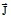and the electric field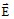, a relationship known as Ohm's law,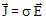.

The electric current resulting from the displacement of free electric charges is called conduction current.

## A perfect conductor…

A perfect conductor is a hypothetical material that offers no resistance to the flow of the electric current. This is a limit case where the electric conductivity σ is infinite.

## Dielectric medium and relative permittivity εr

A dielectric medium is characterized by the following properties:

• the electrons of the atoms are strongly bonded to the nucleus and in effect, free electrons do not exist; consequently, dielectrics are very good electrical insulators.

The value of resistivity ρ = 1/σ is between 1017 and 1019 Ω.m

• in the presence of an electric field:
• the atoms are deformed as a result of the action of the electric field on the clouds of electrons and the nucleus , and
• the arbitrary orientation of molecules ( groups of atoms ) is changed by the action of the electric field on the positive and negative ions

This phenomeno n is called “dielectric polarization” .

The volume density of the moments of electrical dipoles defines the polarization field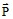. The electric flux density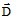, the second quantity characterizing the electric field inside the matter, is defined by the relation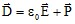, where ε0 is the permittivity of vacuum.

If the medium is characterized by linear polarization, this polarization is proportional to the electric field intensity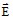. In this case, the relation between the quantitiesandis linear,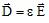, where ε is the permittivity of the material.

In practice, we use the relative permittivity εr, as being the ratio between the permittivity of the material and that of a vacuum εr = ε / ε0. This quantity is also called the dielectric constant of the material.

## A perfect dielectric…

A perfect dielectric is a hypothetical material that does not allow any charge to pass through (a limit case where the conductivity σ is null).

## Displacement electric current

The polarization phenomenon in AC electric fields is responsible for the existence of an AC current, called displacement electric current .

If the electric field varies in time, in sinusoidal mode for example, an oscillatory movement of the dipoles appears because of their tendency to align itself on the electric field. Although these oscillations are local and that there is not thus real migration of the dipoles, this local movement is comparable to a AC current called displacement electric current.

This current is proportional to the product ω.ε between the field pulsation ω =2πf and the permittivity ε.

## Real medium in AC electric fields

The boundary between the dielectric and conducting behavior of a medium in AC electric fields is not absolute, because the matter may be more or less conducting, more or less dielectric.

In fact, a given body is more or less conducting and more or less dielectric according to the frequency. The ratio σ/ωε (ω is the pulsation, ω =2 π f) shows the relative significance of:

• the AC conduction current:

i.e. of the conducting character of the medium (σ),

• and the displacement current:

i.e. of its dielectric character (ω.ε)

Thus, in AC electric field, we have:

a good conductor for σ/ω.ε >> 1,

and a good dielectric for σ/ω.ε << 1.